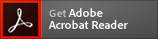Gramicidin from Bacillus aneurinolyticus (Bacillus brevis) A linear polypeptide antibiotic

# Gramicidin from Bacillus aneurinolyticus (Bacillus brevis) (CAS 1405-97-6)

Application: A linear polypeptide antibiotic
CAS Number: 1405-97-6
Molecular Weight: 1882.3
Molecular Formula: C99H140N20O17
* Refer to Certificate of Analysis for lot specific data (including water content).Gramicidin from Bacillus aneurinolyticus (Bacillus brevis) is a linear polypeptide antibiotic with a mixture of gramicidin A, B, C, and D. Gramicidin D is a channel-forming ionophore that flip-flops slowly across the membrane and is a known Pgp substrate and surprisingly was found to inhibit Pgp ATPase activity. This inhibition was reversed by other Pgp substrates suggesting a common drug binding site among MDR substrate-type drugs and chemosensitizers.

#### References

A. Pullman, Energy profiles in the gramicidin A channel, review. Q. Rev. Biophys. 20, 173, (1987) G.A. Brewer, and K. Florey (Eds.) Analytical Profiles of Drug Substances, (1979) 8, 179 Eytan, G.D., et al. J. Biol. Chem. 27, 12897-12902, (1996) Borgnia, M.J., et al. J. Biol. Chem. 27, 3163-3171, (1996)

Appearance :
Powder
Physical State :
Solid
Storage :
Store at 4° C
For Research Use Only. Not Intended for Diagnostic or Therapeutic Use.
RTECS :
MD8225000
PubChem CID :
16130140
MDL Number :
MFCD00131232
EC Number :
215-790-4
SMILES :
C[C@@H](C(=O)N[C@H](CC(C)C)C(=O)N[C@@H](C)C(=O)N[C@H](C(C)C)C(=O)N[C@@H](C(C)C)C(=O)N[C@@H](C(C)C)C(=O)N[C@@H](CC1=CNC2=CC=CC=C21)C(=O)N[C@H](CC(C)C)C(=O)N[C@@H](CC3=CNC4=CC=CC=C43)C(=O)N[C@H](CC(C)C)C(=O)N[C@@H](CC5=CNC6=CC=CC=C65)C(=O)N[C@H](CC(C)C)C(=O)N[C@@H](CC7=CNC8=CC=CC=C87)C(=O)NCCO)NC(=O)CNC(=O)[C@H](C(C)C)NC=O